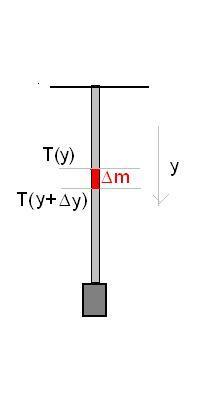# Wave Speed at the top, bottom, and middle of a Hanging Cord

• FunkyFrap

## Homework Statement

A hanging cord is attached to a fixed support at the top and is 78.0m long. It is stretched taut by a weight with mass 21.0kg attached at the lower end. The mass of the cord is 2.20kg . A device at the bottom oscillates the cord by tapping it sideways (Do not neglect the weight of the cord.)

1.) What is the wave speed at the bottom of the cord?

2.)What is the wave speed at the middle of the cord?

3.)What is the wave speed at the top of the cord?

## Homework Equations

$v = \sqrt{T/µ}$
where $T$ is the tension force on the string and $µ$ is the mass per unit length.

## The Attempt at a Solution

After reading my text and browsing the forums for help it seems that the tension of a cord with non-negligible mass in this case is $T = µ(m1 + m2)L$ where $m1$ is the mass of the ball and $m2$ is the mass of the cord.

$T = \sqrt{µ(m1 + m2)*L/µ} = \sqrt{(m1 + m2)*L}$

Plugging in I obtain $42.5 m/s$
Which seems to suggest that the speed is the same at every point. However I'm having serious doubts about the answer I've reached. Is that true? Or am I missing something very important here?

The tension varies at different points along this rope. Consider a point on the rope at a distance x above the bottom of the rope. What must the tension at this point be?

The tension varies at different points along this rope. Consider a point on the rope at a distance x above the bottom of the rope. What must the tension at this point be?

After doing some force analysis, would it make sense to say that $T = m1*g + µ*m2*x?$

After doing some force analysis, would it make sense to say that $T = m1*g + µ*m2*x?$
I think you're on to something, but the dimensions are not correct. $\mu m_2x$ has dimensions of mass squared, but it's supposed to be in units of force.

See a piece of the cord (length Δy and mass Δm=μ Δy). It is acted upon by gravity and the tensions at its top and bottom. The downward forces are balanced by the upward tension T(y) of the upper piece of cord. The downward forces are gravity gΔm and the downward tension T(y+Δy).
T(y)=gΔm + T(y+Δy) or T(y+Δy) -T(y) = - μ Δy. The tension changes evenly along the cord, the slope is constant, T(y) can be represented by a straight line. At the bottom, the cord has to balance the hanging weight only. Write out T(y)...I think you're on to something, but the dimensions are not correct. $\mu m_2x$ has dimensions of mass squared, but it's supposed to be in units of force.
I think I got it now after doing some thinking

At the bottom, only the weight matters, right? so $T = m1*g$
At the middle, we have the weight and half(?) the cord so $T = g*(m1 + 0.5m2)$
At the top, we have the weight and full-cord so $T = g*(m1 + m2)$

Then I can solve for the respective wave speeds
I guess that $T = µ*m*L$ equation is bunk?

I guess that $T = µ*m*L$ equation is bunk?
That is completely wrong. Why do you think it can be correct at all? What is the dimension of the tension? Is it mass-squared?
You know the tensions at top, bottom, and middle already. If you think a bit, you could write out the function T(x).

At the bottom, only the weight matters, right? so $T = m1*g$
At the middle, we have the weight and half(?) the cord so $T = g*(m1 + 0.5m2)$
At the top, we have the weight and full-cord so $T = g*(m1 + m2)$
Right. Essentially, (for the purpose of finding the tension) you can treat the portion of the rope below the point x and the block as a single object. It's weight would be $g(m_1+\mu x)=g(m_1+\frac{x}{L}m_2)$
(x is measured from the bottom)